# 2. Block cross-validation for species distribution modelling

## Introduction

Species distribution models (SDMs) are widely used tools for predicting the potential distribution of a species based on environmental variables. However, it is crucial to evaluate the performance of these models to ensure their accuracy and reliability. One commonly used method for evaluating the performance of SDMs is block cross-validation (read more in Valavi et al. 2019 and the Tutorial 1). This approach allows for a more robust evaluation of the model as it accounts for spatial autocorrelation and other spatial dependencies (Roberts et al. 2017). This document illustrates how to utilize the blockCV package to evaluate the performance of SDMs using block cross-validation.

Two examples are provided: modelling using the randomForest, and biomod2 packages.

Check new updates of blockCV in the tutorial 1- blockCV introduction: how to create block cross-validation folds.

Please cite blockCV by: Valavi R, Elith J, Lahoz-Monfort JJ, Guillera-Arroita G. blockCV: An R package for generating spatially or environmentally separated folds for k-fold cross-validation of species distribution models. Methods Ecol Evol. 2019; 10:225–232. doi: 10.1111/2041-210X.13107

The blockCV package contains the raw format of the following data:

• Raster covariates of Australia (.tif)
• Simulated species data (.csv)

These data are used to illustrate how the package is used. The raster data include several bioclimatic variables for Australia. The species data include presence-absence records (binary) of a simulated species.

To load the package raster data use:

library(sf) # working with spatial vector data
library(terra) # working with spatial raster data
library(tmap) # plotting maps

# the pipe operator |> is available for R version 4.1 or higher
rasters <- system.file("extdata/au/", package = "blockCV") |>
list.files(full.names = TRUE) |>
terra::rast()

The presence-absence species data include 243 presence points and 257 absence points.

# load species presence-asence data and convert to sf
points <- read.csv(system.file("extdata/", "species.csv", package = "blockCV"))
head(points)
##            x        y occ
## 1  1313728.4 -2275453   0
## 2  1176795.0 -1916003   0
## 3 -1741599.3 -3927213   1
## 4  1099769.9 -4124055   1
## 5  1279495.1 -3901538   0
## 6   928603.1 -1342594   0

The appropriate format of species data for the blockCV package is simple features (from the sf package). The data is provide in GDA2020 / GA LCC coordinate reference system with "EPSG:7845" as defined by crs = 7845. We convert the data.frame to sf as follows:

pa_data <- sf::st_as_sf(points, coords = c("x", "y"), crs = 7845)

Let’s plot the species data using tmap package:

tm_shape(rasters[]) +
tm_raster(legend.show = FALSE, n = 30, palette = gray.colors(10)) +
tm_shape(pa_data) +
tm_dots(col = "occ", style = "cat", size = 0.1)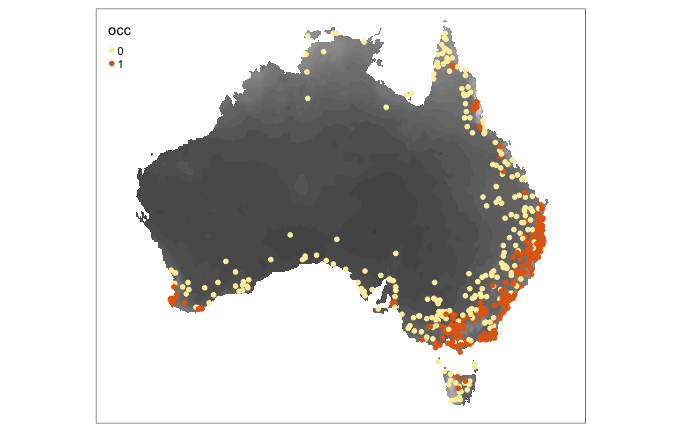# Generating block CV folds

Here, we generate two CV strategies, one k-fold CV using cv_spatial and one LOO CV using cv_nndm. See more options and configurations in the Tutorial 1 - introduction to blockCV.

library(blockCV)

Creating spatial blocks:

scv1 <- cv_spatial(
x = pa_data,
column = "occ", # the response column (binary or multi-class)
r = rasters,
k = 5, # number of folds
size = 360000, # size of the blocks in metres
selection = "random", # random blocks-to-fold
iteration = 50, # find evenly dispersed folds
progress = FALSE, # turn off progress bar
biomod2 = TRUE, # also create folds for biomod2
raster_colors = terrain.colors(10, rev = TRUE) # options from cv_plot for a better colour contrast
) 
##
##   train_0 train_1 test_0 test_1
## 1     198     168     59     75
## 2     210     212     47     31
## 3     212     179     45     64
## 4     189     200     68     43
## 5     219     213     38     30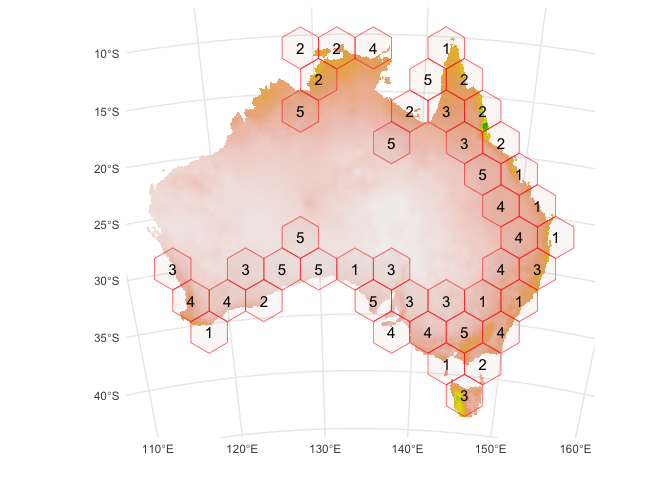Now, let’s create LOO CV folds with nearest neighbour distance matching (NNDM; Milà et al. 2022) algorithm. To run cv_nndm, you need a measure of spatial autocorrelation present in your data. This can be done wither by 1) fitting a model and use it’s residual to calculate spatial autocorrelation, or 2) use the autocorrelation of response variable for it (Milà et al, 2022; Roberts et al. 2017). Here, we calculate spatial autocorrelation range in the response using the cv_spatial_autocor function.

range <- cv_spatial_autocor(
x = pa_data, # species data
column = "occ", # column storing presence-absence records (0s and 1s)
plot = FALSE
)

range$range ##  362036 So the range of spatial autocorrelation is roughly 360 kilometres. scv2 <- cv_nndm( x = pa_data, column = "occ", r = rasters, size = 360000, # range of spatial autocorrelation num_sample = 10000, # number of samples of prediction points sampling = "regular", # sampling methods; it can be random as well min_train = 0.1, # minimum portion to keep in each train fold plot = TRUE ) ## train_0 train_1 test_0 test_1 ## Min. :210.0 Min. :145.0 Min. :0.000 Min. :0.000 ## Mean :244.4 Mean :221.5 Mean :0.514 Mean :0.486 ## Max. :257.0 Max. :243.0 Max. :1.000 Max. :1.000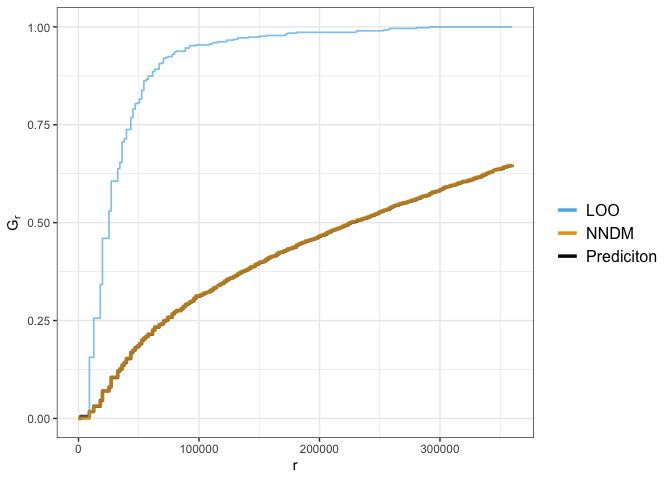You can visualise the generated folds of both methods using cv_plot function. Here is three folds from the cv_nndm object: # see the number of folds in scv2 object scv2$k
##  500
cv_plot(
cv = scv2, # cv object
x = pa_data, # species spatial data
num_plots = c(1, 10, 100) # three of folds to plot
)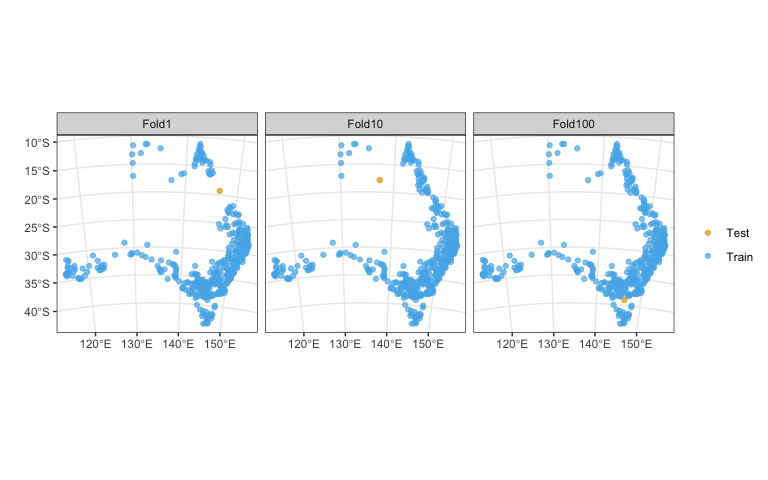## Evaluating SDMs with block cross-validation: examples

In this section, we show how to use the folds generated by blockCV in the previous sections for the evaluation of SDMs constructed on the species data available in the package. The blockCV stores training and testing folds in three different formats. The common format for all three blocking strategies is a list of the indices of observations in each fold. For cv_spatial and cv_cluster (but not cv_buffer and cv_nndm), the folds are also stored in a matrix format suitable for the biomod2 package and a vector of fold’s number for each observation. This is equal to the number of observation in species spatial data. These three formats are stored in the cv objects as folds_list, biomod_table and folds_ids respectively.

### Using blockCV with Random Forest model

Folds generated by cv_nndm function are used here (a training and testing fold for each record) to show how to use folds from this function (the cv_buffer is also similar to this approach) for evaluation species distribution models.

Note that with cv_nndm using presence-absence data (and any other type of data except for presence-background data when presence_bg = TRUE is used), there is only one point in each testing fold, and therefore AUC cannot be calculated for each fold separately. Instead, the value of each point is first predicted to the testing point (of each fold), and then a unique AUC is calculated for the full set of predictions.

# loading the libraries
library(randomForest)
library(precrec)

# extract the raster values for the species points as a dataframe
model_data <- terra::extract(rasters, pa_data, df = TRUE, ID = FALSE)
# adding species column to the dataframe
model_data$occ <- as.factor(pa_data$occ)

# extract the fold indices from buffering object
# created in the previous section
# the folds_list works for all four blocking strategies
folds <- scv2$folds_list # create a data.frame to store the prediction of each fold (record) test_table <- pa_data test_table$preds <- NA

for(k in seq_len(length(folds))){
# extracting the training and testing indices
# this way works with folds_list list (but not folds_ids)
trainSet <- unlist(folds[[k]]) # training set indices; first element
testSet <- unlist(folds[[k]]) # testing set indices; second element
rf <- randomForest(occ ~ ., model_data[trainSet, ], ntree = 500) # model fitting on training set
test_table$preds[testSet] <- predict(rf, model_data[testSet, ], type = "prob")[,2] # predict the test set } # calculate Area Under the ROC and PR curves and plot the result precrec_obj <- evalmod(scores = test_table$preds, labels = test_table$occ) auc(precrec_obj) ## modnames dsids curvetypes aucs ## 1 m1 1 ROC 0.8798258 ## 2 m1 1 PRC 0.8734455 Plot the curves: library(ggplot2) autoplot(precrec_obj)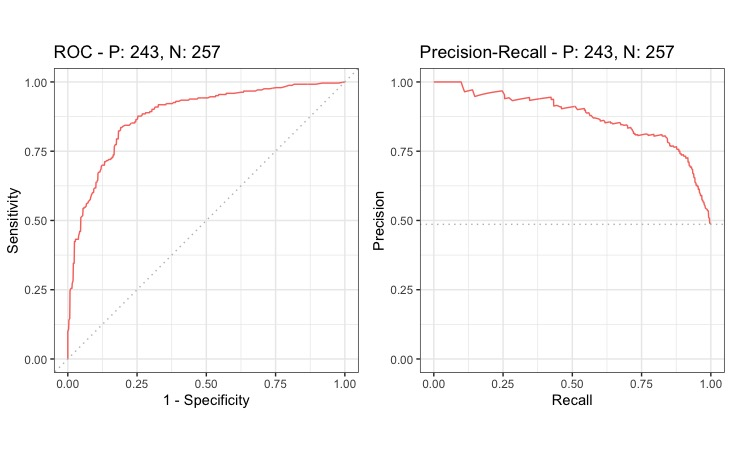### Using blockCV in biomod2 package Package biomod2 (Thuiller et al., 2017) is a commonly used platform for modelling species distributions in an ensemble framework. In this example, we show how to use blockCV folds in biomod2. In this example, the data.split.table generated by cv_spatial is used to evaluate three modelling methods implemented in biomod2. The data.split.table can be generated by both cv_spatial and cv_cluster functions and it is stored as biomod_table in their output objects. # loading the library library(biomod2) # extract the raster values for the species points as a dataframe raster_values <- terra::extract(rasters, pa_data, df = TRUE, ID = FALSE) # 1. Formatting Data biomod_data <- BIOMOD_FormatingData(resp.var = pa_data$occ,
expl.var = raster_values,
resp.xy = sf::st_coordinates(pa_data),
resp.name = "occ",
na.rm = TRUE)

# 2. Defining the folds for data.split.table
# note that biomod_table should be used here not folds
# use generated folds from cv_spatial in previous section
spatial_cv_folds <- scv1\$biomod_table

# 3. Defining Models Options; using default options here.
biomod_options <- BIOMOD_ModelingOptions()

# 4. Model fitting
biomod_model_out <- BIOMOD_Modeling(biomod_data,
models = c('GLM','MARS','GBM'),
bm.options = biomod_options,
data.split.table = spatial_cv_folds,
var.import = 0,
metric.eval = c('ROC'),
do.full.models = TRUE)
# 5. Model evaluation
biomod_model_eval <- get_evaluations(biomod_model_out)
biomod_model_eval[c("run", "algo", "metric.eval", "calibration", "validation")]
##     run algo metric.eval calibration validation
## 1  RUN1  GLM         ROC       0.921      0.878
## 2  RUN1 MARS         ROC       0.927      0.868
## 3  RUN1  GBM         ROC       0.972      0.914
## 4  RUN2  GLM         ROC       0.911      0.952
## 5  RUN2 MARS         ROC       0.916      0.941
## 6  RUN2  GBM         ROC       0.970      0.928
## 7  RUN3  GLM         ROC       0.921      0.889
## 8  RUN3 MARS         ROC       0.926      0.886
## 9  RUN3  GBM         ROC       0.974      0.899
## 10 RUN4  GLM         ROC       0.934      0.808
## 11 RUN4 MARS         ROC       0.941      0.833
## 12 RUN4  GBM         ROC       0.976      0.836
## 13 RUN5  GLM         ROC       0.906      0.934
## 14 RUN5 MARS         ROC       0.917      0.871
## 15 RUN5  GBM         ROC       0.973      0.886

The validation column shows the result of spatial cross-validation and each RUN is a CV fold.